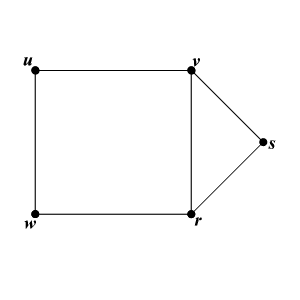# Graph Theory

In the branch of mathematics called graph theory, some words have different definitions.

In graph theory, a graph  is a set of objects called vertices (or nodes) connected by links called edges . (It's not the same kind of graph you draw when you graph a function on coordinate axes.)

This kind of graph is sometimes also called a network

A finite simple graph is an ordered pair $G=\left[V,E\right]$ , where $V$ is a finite set of vertices or nodes and each element of $E$ is a subset of $V$ with exactly $2$ elements. Typically, a graph is depicted as a set of dots (the vertices) connected by lines (the edges).

The order of a graph is | $V$ | (the number of vertices). A graph's size is | $E$ | , the number of edges. The degree of a vertex is the number of edges that connect to it.

Example:In the above graph, the set of vertices are $V=\left\{u,v,w,r,s\right\}$ and the set of edges are $E=\left\{uv,uw,wr,vr,rs,vs\right\}$ .

The order of the graph is $5$ . The size of the graph is $6$ .

The number of edges that connect with vertex $u$ is $2$ and therefore the degree of the vertex $u$ is $2$ .

 Vertices Degree $u$ 2 $v$ 3 $w$ 2 $r$ 3 $s$ 2